# 使用 Win2D 实现融合效果

## 1. 融合效果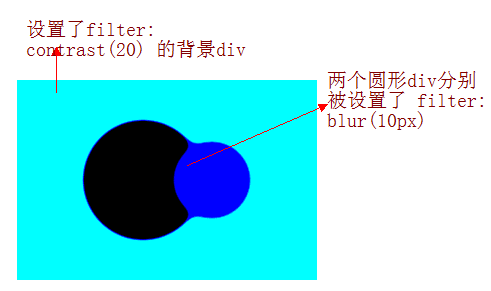## 2. 使用 Win2D 实现融合效果

Win2D 是一个很简单使用的底层图形 Windows Runtime API。它利用了 Direct2D 的强大功能，并与 UWP 和 WinUI 3 的 Xaml 无缝集成，可以使用强大的渲染得到漂亮界面。UWP 和 WinUI 3 版本的 Nuget 安装脚本分别为：

Install-Package Win2D.uwp -Version 1.26.0

Install-Package Microsoft.Graphics.Win2D -Version 1.0.3.1

private ICanvasBrush _leftBrush;
private ICanvasBrush _rightBrush;
private ICanvasImage _image;
private GaussianBlurEffect _blurEffect;

private void OnCreateResource(CanvasControl sender, CanvasCreateResourcesEventArgs args)
{
_leftBrush = new CanvasSolidColorBrush(sender, Colors.Black);
_rightBrush = new CanvasSolidColorBrush(sender, Colors.Blue);
_blurEffect = new GaussianBlurEffect
{
BlurAmount = 20f
};

_image = new ColorMatrixEffect
{
ColorMatrix = new Matrix5x4
{
M11 = 1,
M12 = 0,
M13 = 0,
M14 = 0,
M21 = 0,
M22 = 1,
M23 = 0,
M24 = 0,
M31 = 0,
M32 = 0,
M33 = 1,
M34 = 0,
M41 = 0,
M42 = 0,
M43 = 0,
M44 = 18,
M51 = 0,
M52 = 0,
M53 = 0,
M54 = -7
},
Source = _blurEffect
};
}


private void OnCanvasSizeChanged(object sender, SizeChangedEventArgs e)
{
_centerPoint = Canvas.ActualSize / 2;
}


private void OnDraw(CanvasControl sender, CanvasDrawEventArgs args)
{
var source = new CanvasCommandList(sender);
using (var drawingSession = source.CreateDrawingSession())
{
drawingSession.FillCircle(_centerPoint + new Vector2(-75, 0), 100, _leftBrush);
drawingSession.FillCircle(_centerPoint + new Vector2(75, 0), 60, _rightBrush);
}

_blurEffect.Source = source;
args.DrawingSession.DrawImage(_image);
}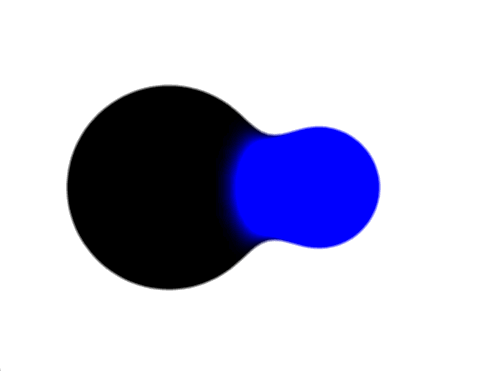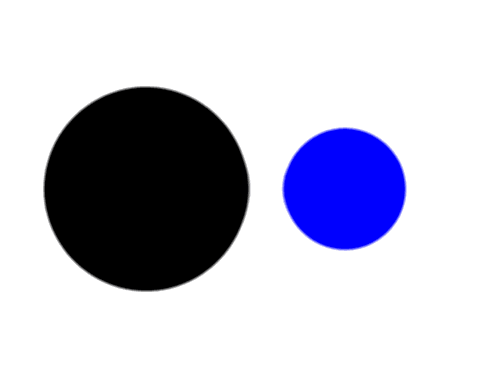## 3. Win2D 中融合效果的原理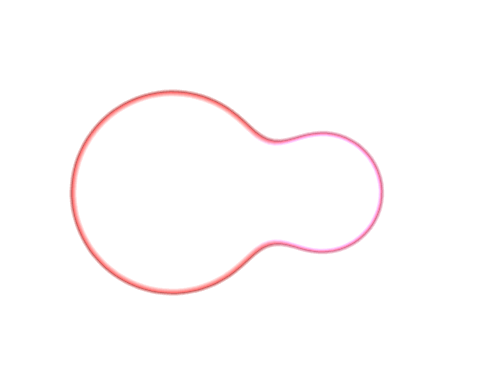### 3.1 GaussianBlurEffect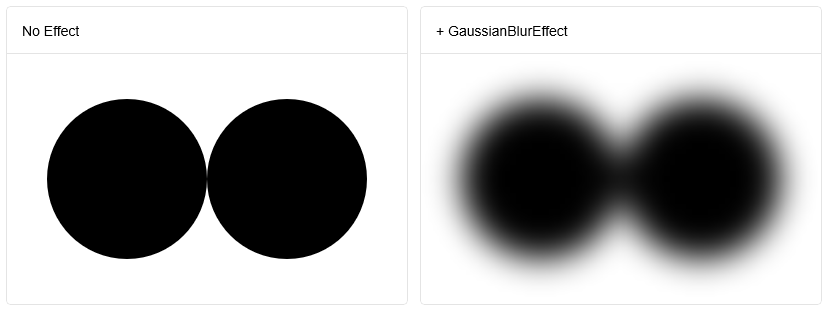### 3.2 ColorMatrixEffect

ColorMatrixEffect 使用 Matrix5x4 修改图像的颜色。这个 Matrix5x4 的默认值是：

var identity = new Matrix5x4
{
M11 = 1, M12 = 0, M13 = 0, M14 = 0,
M21 = 0, M22 = 1, M23 = 0, M24 = 0,
M31 = 0, M32 = 0, M33 = 1, M34 = 0,
M41 = 0, M42 = 0, M43 = 0, M44 = 1,
M51 = 0, M52 = 0, M53 = 0, M54 = 0
};


result.R = (src.R * matrix.M11) + (src.G * matrix.M21) + (src.B * matrix.M31) + (src.A * matrix.M41) + matrix.M51
result.G = (src.R * matrix.M12) + (src.G * matrix.M22) + (src.B * matrix.M32) + (src.A * matrix.M42) + matrix.M52
result.B = (src.R * matrix.M13) + (src.G * matrix.M23) + (src.B * matrix.M33) + (src.A * matrix.M43) + matrix.M53
result.A = (src.R * matrix.M14) + (src.G * matrix.M24) + (src.B * matrix.M34) + (src.A * matrix.M44) + matrix.M54


result.A =  (src.A * 18) -7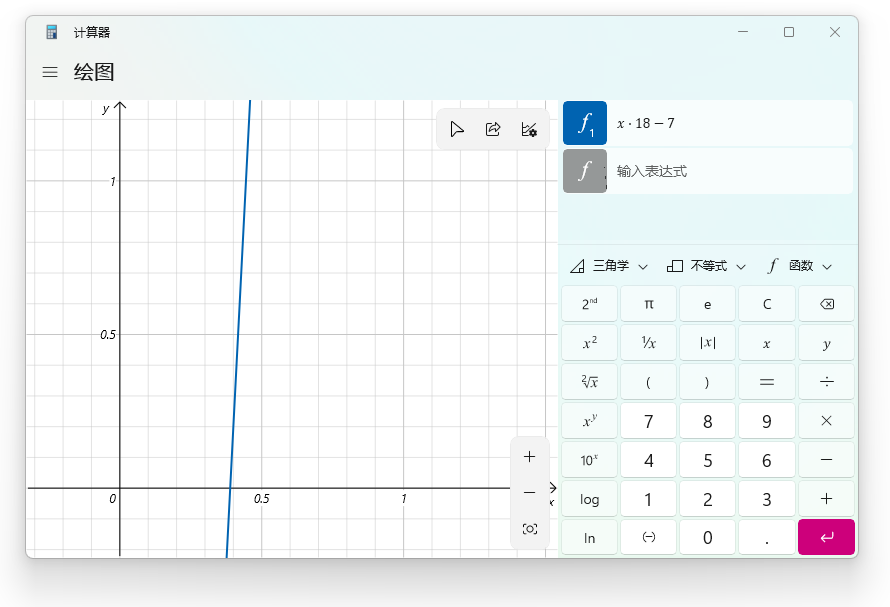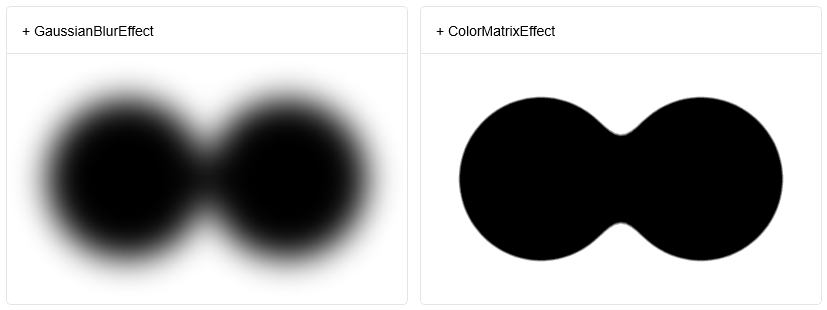### 3.3 ClampOutput

result.R = source.R * source.A;
result.G = source.G * source.A;
result.B = source.B * source.A;
result.A = source.A;


Win2D: Premultiplied alpha

## 4. 最后## 6. 参考

cnbluefire_GooeyButton

https://github.com/Microsoft/Win2D

ColorMatrixEffect Class

GaussianBlurEffect Class

posted @ 2022-09-22 08:59  dino.c  阅读(2509)  评论(7编辑  收藏  举报